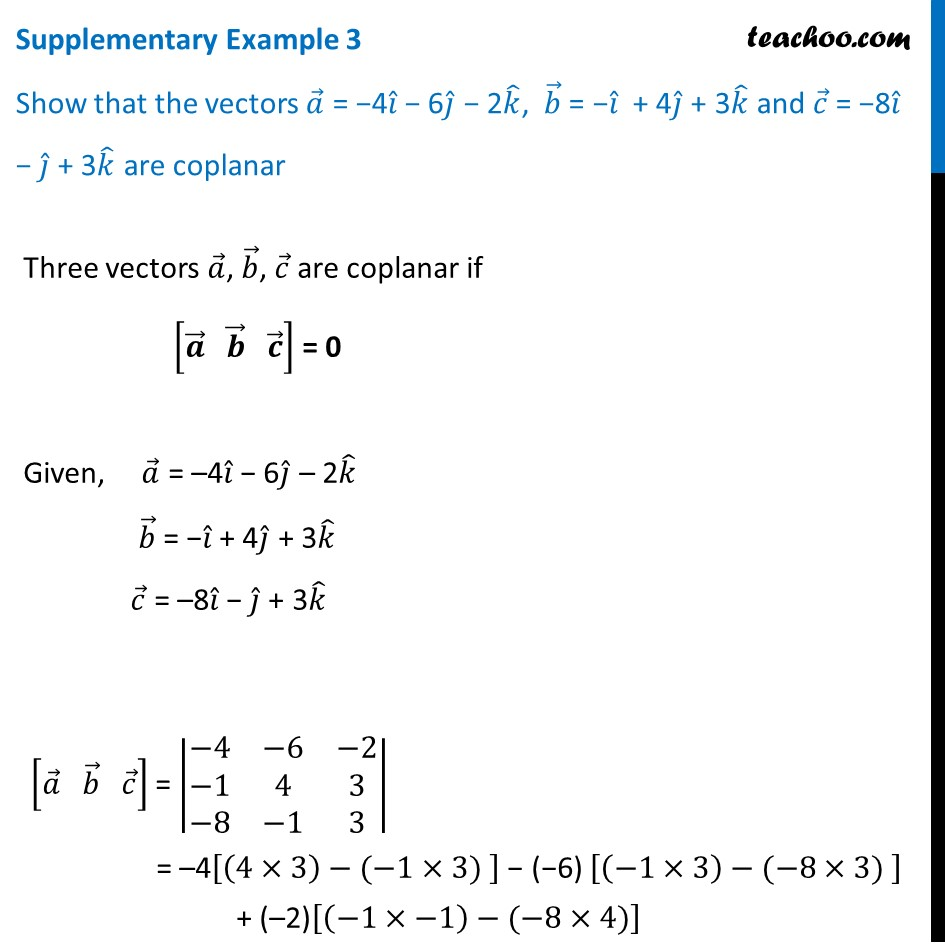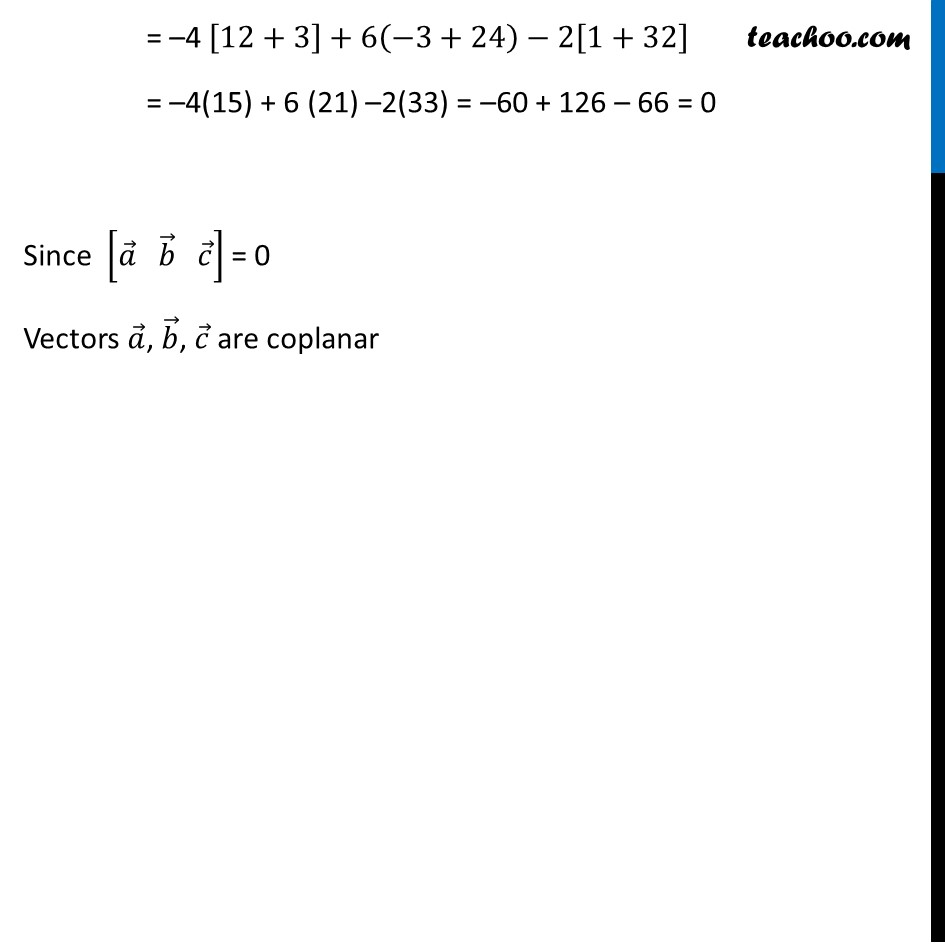Supplementary examples and questions from CBSE

Chapter 10 Class 12 Vector Algebra
Serial order wiseLearn in your speed, with individual attention - Teachoo Maths 1-on-1 Class

### Transcript

Supplementary Example 3 Show that the vectors 𝑎 ⃗ = −4𝑖 ̂ − 6𝑗 ̂ − 2𝑘 ̂, 𝑏 ⃗ = −𝑖 ̂ + 4𝑗 ̂ + 3𝑘 ̂ and 𝑐 ⃗ = −8𝑖 ̂ − 𝑗 ̂ + 3𝑘 ̂ are coplanar Three vectors 𝑎 ⃗, 𝑏 ⃗, 𝑐 ⃗ are coplanar if [𝒂 ⃗" " 𝒃 ⃗" " 𝒄 ⃗ ] = 0 Given, 𝑎 ⃗ = –4𝑖 ̂ − 6𝑗 ̂ – 2𝑘 ̂ 𝑏 ⃗ = −𝑖 ̂ + 4𝑗 ̂ + 3𝑘 ̂ 𝑐 ⃗ = –8𝑖 ̂ − 𝑗 ̂ + 3𝑘 ̂ [𝑎 ⃗" " 𝑏 ⃗" " 𝑐 ⃗ ] = |■8(−4&−6&−2@−1&4&3@−8&−1&3)| = –4[(4×3)−(−1×3) ] − (−6) [(−1×3)−(−8×3) ] + (–2)[(−1×−1)−(−8×4)] = –4 [12+3]+6(−3+24)−2[1+32] = –4(15) + 6 (21) –2(33) = –60 + 126 – 66 = 0 Since [𝑎 ⃗" " 𝑏 ⃗" " 𝑐 ⃗ ] = 0 Vectors 𝑎 ⃗, 𝑏 ⃗, 𝑐 ⃗ are coplanar